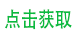## django 获取字段选项 choice 的值

choices

```YEAR_IN_SCHOOL_CHOICES = (
('FR', 'Freshman'),
('SO', 'Sophomore'),
('JR', 'Junior'),
('SR', 'Senior'),
)
```

```from django.db import models

class Person(models.Model):
SHIRT_SIZES = (
('S', 'Small'),
('M', 'Medium'),
('L', 'Large'),
)
name = models.CharField(max_length=60)
shirt_size = models.CharField(max_length=1, choices=SHIRT_SIZES)
```
```>>> p = Person(name="Fred Flintstone", shirt_size="L")
>>> p.save()
>>> p.shirt_size
'L'
>>> p.get_shirt_size_display()
'Large'```

``````class Model(six.with_metaclass(ModelBase)):
# ....

def _get_FIELD_display(self, field):
value = getattr(self, field.attname)
return force_text(dict(field.flatchoices).get(value, value), strings_only=True)``````

^_^